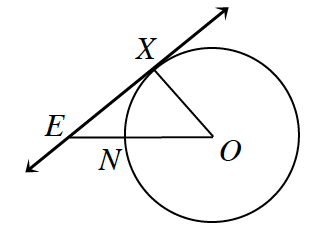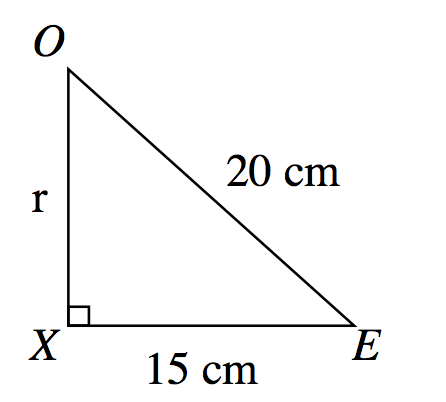### Home > INT2 > Chapter 11 > Lesson 11.1.2 > Problem11-23

11-23.In the figure at right, $EX$ is tangent to $⊙O$ at point $X$. $OE = 20.0$ cm and $XE = 15.0$ cm.

1. What is the area of the circle?

First find the length of the radius $(XO)$ by using the pythagorean theorem. Refer to the figure at right for help visualizing the problem.

Area $= 175π$ cm$^2$2. What is the area of the sector bounded by $\overline{OX}$ and $\overline{ON}$?

$\text{Area of sector} \overset{\frown}{NX}=\frac{\angle O}{360^{\circ}}(175\pi)$

Use trig identities to help you find $∠O$.

3. What is the area of the region bounded by $XE, NE,$ and $\overarc { N X }$?

Subtract the area of the sector you found in part (b) from the area of $ΔOEX$.